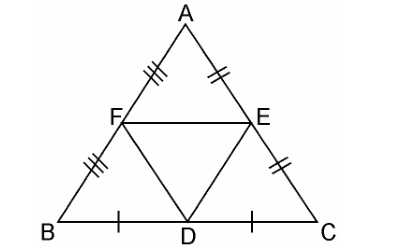# D, E and F are respectively the mid-points of sides AB, BC and CA of ΔABC. Find the ratio of the area of ΔDEF and ΔABC.

Given

D, E and F are respectively the mid-points of sides AB, BC and CA of ΔABC.

Find out

We have to find the ratio of the area of ΔDEF and ΔABC.In ΔABC, given that

F is the mid-point of AB

E is the mid-point of AC

So, by the mid-point theorem, we get to know

FE || BC and FE = 1/2BC

⇒ FE || BC and FE || BD [BD = 1/2BC]

Since, opposite sides of parallelogram are equal and parallel

∴ BDEF is parallelogram.

Furthermore, in ΔFBD and ΔDEF, we have

FB = DE (Opposite sides of parallelogram BDEF)

FD = FD (Common sides)

BD = FE (Opposite sides of parallelogram BDEF)

∴ ΔFBD ≅ ΔDEF

Thus, we can prove that

ΔAFE ≅ ΔDEF

ΔEDC ≅ ΔDEF

We know that triangles are congruent, then they are equal in area.

So,

Area(ΔFBD) = Area(ΔDEF) ……………………………(i)

Area(ΔAFE) = Area(ΔDEF) ……………………………….(ii)

and,

Area(ΔEDC) = Area(ΔDEF) ………………………….(iii)

Now,

Area(ΔABC) = Area(ΔFBD) + Area(ΔDEF) + Area(ΔAFE) + Area(ΔEDC) ………(iv)

Area(ΔABC) = Area(ΔDEF) + Area(ΔDEF) + Area(ΔDEF) + Area(ΔDEF)

From equation (i), (ii) and (iii),

⇒ Area(ΔDEF) = (1/4)Area(ΔABC)

⇒ Area(ΔDEF)/Area(ΔABC) = 1/4

Hence, Area(ΔDEF): Area(ΔABC) = 1:4